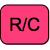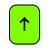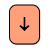Wisdom of the Crowd Health Check Report Classifier 1 2 3 4 α Labelβ LabelClassifier ok, agreeing with most, more than 50%.Classifier flipped, disagreeing with most, less than 50%.Classifier numbers either random or too correlated for this tool.

# How Wise is Your Crowd?

Check the health of your 4 classifier ensemble. Is one of them inverted?
 α,α,α,α A positive integer
 β,β,β,β A positive integer
 β,α,α,α A positive integer
 α,β,β,β A positive integer
 α,β,α,α A positive integer
 β,α,β,β A positive integer
 α,α,β,α A positive integer
 β,β,α,β A positive integer
 α,α,α,β A positive integer
 β,β,β,α A positive integer
 α,α,β,β A positive integer
 β,β,α,α A positive integer
 β,α,α,β A positive integer
 α,β,β,α A positive integer
 α,β,α,β A positive integer
 β,α,β,α A positive integer